Question

# 31. What is ∆G for a reaction where ∆G° = -4.5 kJ/mol and Q = 4.0...

31. What is ∆G for a reaction where ∆G° = -4.5 kJ/mol and Q = 4.0 at 295 K? (R = 8.314 J/mol ･ K)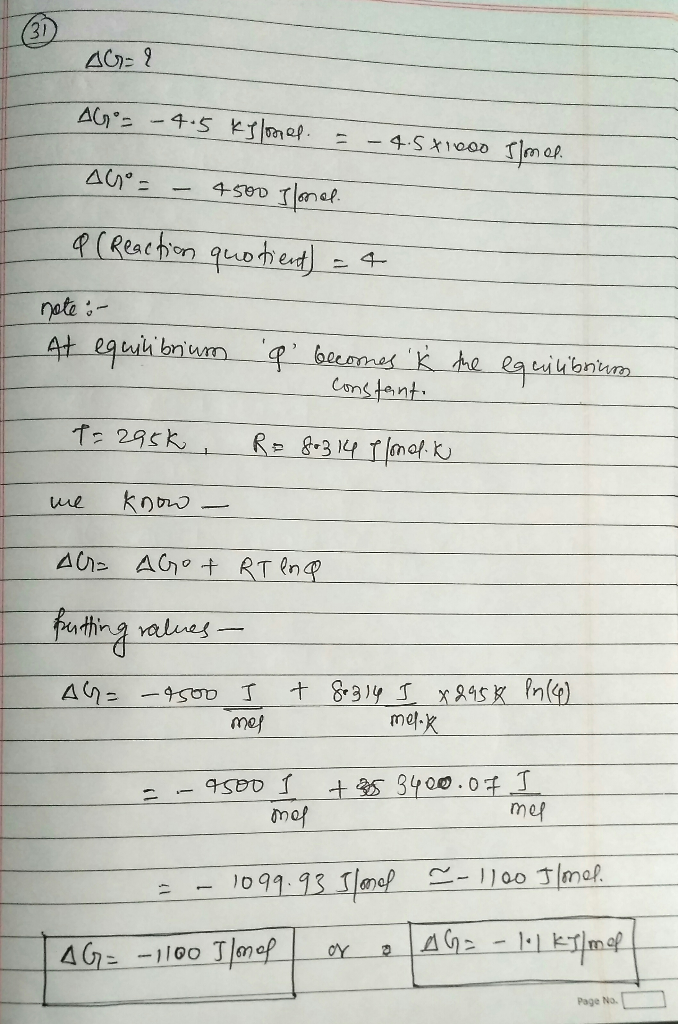∆G=-1100J/mol or -1.1kj/mol

#### Earn Coins

Coins can be redeemed for fabulous gifts.

Similar Homework Help Questions
• ### Determine AGⓇ for a reaction when AG = -184.7 kJ/mol and Q = 0.043 at 298...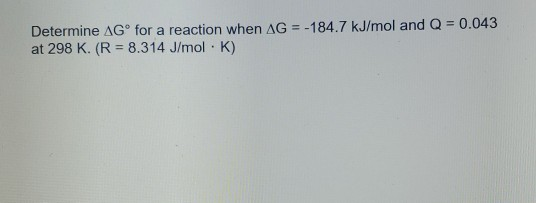Determine AGⓇ for a reaction when AG = -184.7 kJ/mol and Q = 0.043 at 298 K. (R = 8.314 J/mol K)

• ### Determine Gº for a reaction when AG = -119.1 kJ/mol and Q = 0.043 at 298...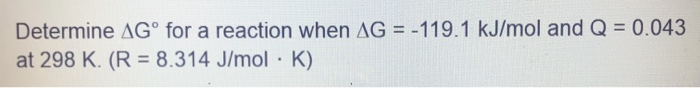Determine Gº for a reaction when AG = -119.1 kJ/mol and Q = 0.043 at 298 K. (R = 8.314 J/mol · K)

• ### AG°= AH-TAS AG=AGº+RTinQ where R=8.314 J/mol K 1. Calculate AGº for the following reaction at 25 °C if AH°= -1854 kJ...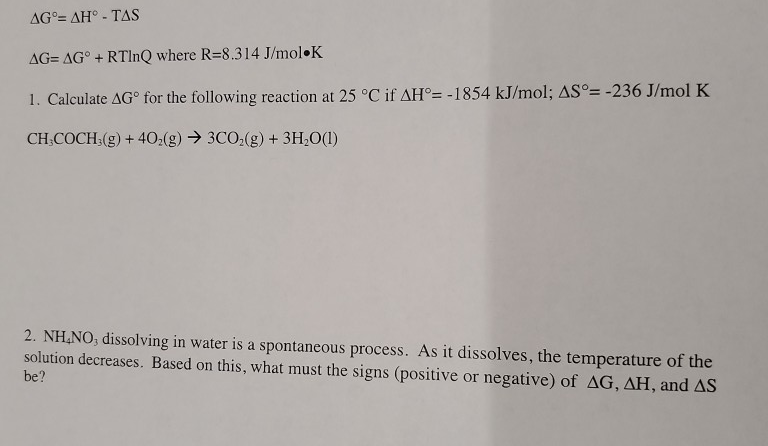AG°= AH-TAS AG=AGº+RTinQ where R=8.314 J/mol K 1. Calculate AGº for the following reaction at 25 °C if AH°= -1854 kJ/mol; AS°= -236 J/mol K CH-COCH3(g) + 402(g) → 3C02(g) + 3H2O(1) 2. NH.NO, dissolving in water is a spontaneous process. As it dissolves, the temperature of the solution decreases. Based on this, what must the signs (positive or negative) of AG, AH, and AS be?

• ### Determine K for a reaction at 200 Kif AG° = 27.8 kJ/mol. (R = 8.314 J/mol...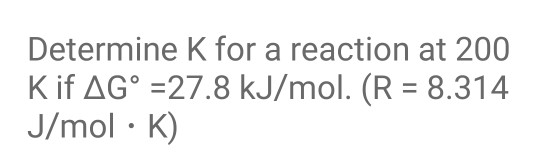Determine K for a reaction at 200 Kif AG° = 27.8 kJ/mol. (R = 8.314 J/mol · K)

• ### Determine K for a reaction at 200 K if AG° = 12.9 kJ/mol. (R = 8.314...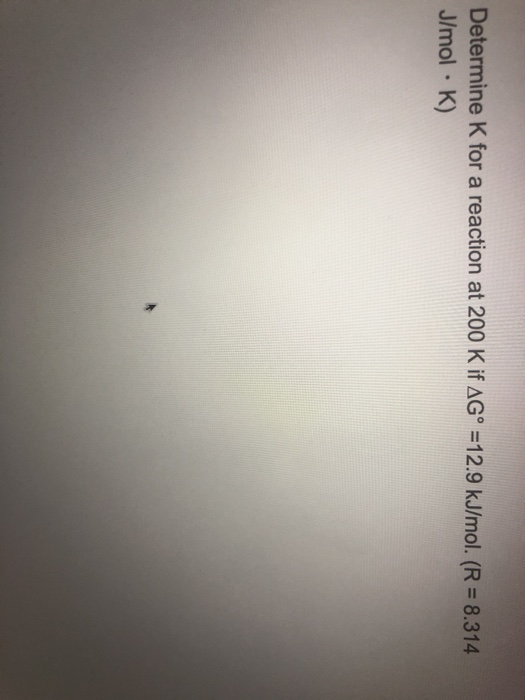Determine K for a reaction at 200 K if AG° = 12.9 kJ/mol. (R = 8.314 J/mol · K)

• ### For a given reaction, the activation energy is 61.5 kJ/mol. If the reaction rate constant is...

For a given reaction, the activation energy is 61.5 kJ/mol. If the reaction rate constant is 5.23E-3 M-1s-1 at 38.0 °C, what is the reaction rate constant at -3.00 °C? (R = 8.314 J/K×mol)

• ### The activation energy, Ea, for a particular reaction is 19.4 kJ/mol. If the rate constant at...

The activation energy, Ea, for a particular reaction is 19.4 kJ/mol. If the rate constant at 80 °C is 0.820 M⁻¹s⁻¹, then what is the value of the rate constant at 209 °C? (R = 8.314 J/mol • K)

• ### The activation energy, Ea, for a particular reaction is 19.4 kJ/mol. If the rate constant at...

The activation energy, Ea, for a particular reaction is 19.4 kJ/mol. If the rate constant at 80 °C is 0.820 M⁻¹s⁻¹, then what is the value of the rate constant at 121 °C? (R = 8.314 J/mol • K)

• ### A certain reaction has a ΔG value of 13.8 kJ/mol. What is the value of the...

A certain reaction has a ΔG value of 13.8 kJ/mol. What is the value of the equilibrium constant (K) for this reaction when the temperature is 295 K? What is the value of K at 305 K when ∆Go = -4.75 kJ/mol? Consider a process with ∆H = 37.6 kJ and ∆S = 99.5 J/K. At what temperature will this process be at equilibrium?

• ### QUESTION 19 Calculate AG, in kJ, for the following reaction: 2 HNO, (lia) + NO (g)...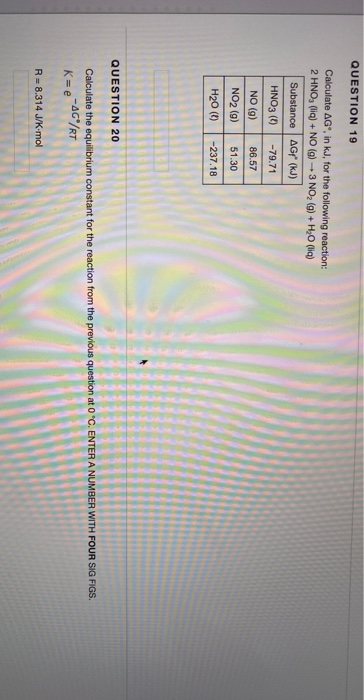QUESTION 19 Calculate AG, in kJ, for the following reaction: 2 HNO, (lia) + NO (g) - 3 NO, (g) + H2O (lia) Substance AG (kJ) HNO3 () - 79.71 NO (9) 86.57 51.30 NO2 (9) H20 (0) -237.18 QUESTION 20 Calculate the equilibrium constant for the reaction from the previous question at O "C. ENTER A NUMBER WITH FOUR SIG FIGS. K=e-4CRT R = 8.314 J/K mol